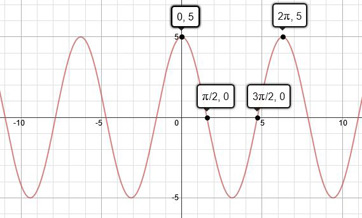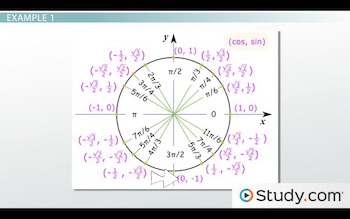# Solving Trigonometric Equations with Restricted Domains

An error occurred trying to load this video.

Try refreshing the page, or contact customer support.

Coming up next: Solving Trigonometric Equations with Infinite Solutions

### You're on a roll. Keep up the good work!

Replay
Your next lesson will play in 10 seconds
• 0:02 Trigonometric Equations
• 0:57 Restricted Domains
• 2:17 Example 1
• 4:16 Example 2
• 6:07 Lesson Summary
Save Save

Want to watch this again later?

Timeline
Autoplay
Autoplay
Speed Speed Audio mode

#### Recommended Lessons and Courses for You

Lesson Transcript
Instructor: Yuanxin (Amy) Yang Alcocer

Amy has a master's degree in secondary education and has taught math at a public charter high school.

After watching this video lesson, you will be able to find the right answer for a trigonometric equation with a restricted domain. Learn what it means to have a restricted domain and what you need to do to find your answer.

## Trigonometric Equations

In this video lesson, we will look at solving trigonometric equations. These are our equations involving the trigonometric functions. So, our variable will be part of the trig function's argument. Do you remember your trig functions? We have our basic ones of sine, cosine, and tangent. We also have cosecant, secant, and cotangent.

So, our trigonometric equations will have at least one of these functions in the equation, such as f(x) = sin (x) + 2. It could even have a combination of trig functions such as f(x) = cos (x) + cot (x). Notice how our variable is part of the argument in both of these equations? This is what makes these equations trigonometric equations.

## Restricted Domains

In this video lesson, we do have one additional criteria that we are adding onto our trigonometric equations. And this is called a restricted domain. What does this mean? It means that we are limiting the possible values that our variable can be. Because we are working with trigonometric equations, our variable will be in radian units. So, you will most likely see a pi in the restricted domain.

For example, you might see f(x) = sin (x) + 2; 0 < x < pi.

This tells you that if you are solving this function, then you are looking for the solution that lies between 0 and pi. Remember that our trigonometric equations are all periodic, which means that the solutions repeat every so often. For sine and cosine, the standard period is 2pi. For tangent, it is pi. Remember that your calculator only gives you the primary solution and you might have to do some additional calculations to find the right answer that is within the restricted domain. Sometimes, if our domain is too restrictive, though, we may not have a solution at all. Let's take a look at a couple of examples.

## Example 1

Solve f(x) = 5 cos (x); 0 <= x <= 2pi.

This problem is telling us to find the solutions of f(x) = 5 cos (x). This we can easily do. But wait, it also tells us that our answers are limited to between 0 and 2pi inclusive. So, this means that when we find our answers, we have to make sure they are within this range. Let's see what comes of this.

Solving for x means we set our equation to 0. We get 5 cos (x) = 0. To solve for x, we divide by 5 on both sides and then take an arccosine. We get x = cos^-1 (0). What does this equal? Our calculator tells us that it equals 90 degrees or pi/2 radians. Are we done though? Have we found all the answers? Let's graph out our function to see:I have marked our restricted domain by labeling the x = 0 point and the x = 2pi point. We just look between these two points to see how many times our graph crosses the x axis. We see that it crosses the x axis twice between these two points. That means that we have a total of two answers. We found our first one, pi/2. So, what is the second one?

I have already labeled it on this graph. But if this point isn't labeled for you, then you can refer to your unit circle to help you find it. Looking at our unit circle, we see that another point where cos (x) is equal to 0 is when x = 3pi/2:So, our two answers are pi/2 and 3pi/2. Both of these answers are within the limits of our domain, so both are valid answers. And now we are done.

## Example 2

Let's take a look at one more.

Solve f(x) = sin (x) - 1; 2pi < x < 3pi.

To unlock this lesson you must be a Study.com Member.

### Register to view this lesson

Are you a student or a teacher?

#### See for yourself why 30 million people use Study.com

##### Become a Study.com member and start learning now.
Back
What teachers are saying about Study.com

### Earning College Credit

Did you know… We have over 200 college courses that prepare you to earn credit by exam that is accepted by over 1,500 colleges and universities. You can test out of the first two years of college and save thousands off your degree. Anyone can earn credit-by-exam regardless of age or education level.